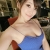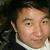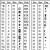# JS Array 中 shift 和 pop 的妙用

```var arr = ['s','o','f','i','s','h'];

arr.shift(); // 返回 's'
arr; // 目前是 ['o','f','i','s','h']
arr.pop() // 返回 'h'
arr // 目前是 ['o','f','i','s']```

### 一、使用 `shift`

```// fn 的作用域限定于 object 下
// 除 object 外，所有 bind 方法的参数都将传给 fn
fn.bind(object, param1, param2, [, paramN]);```

```// 来自 Prototype.js 的 [`.bind`](http://www.prototypejs.org/api/function/bind) 方法
Function.prototype.bind = function(){
var fn = this,
args = Array.prototype.slice.call(arguments),
object = args.shift();
return function(){
return fn.apply(object,
args.concat(Array.prototype.slice.call(arguments)));
};
};```

### 二、使用 `pop`

`define(id, dependencies, callback)`

```var define = function(){
// 取出这个 callback
var args = [].slice.call(arguments)
fn = args.pop();
// 做点其他神马事
fn.apply(null, args)
// ...
},
callback = function(){
var args = arguments, i = 0, len = args.length;
if(len === 0) console.log('只有一个 callback');
for(;i<len;i++) {
console.log(args[i]);
}
}

// 看看他们三个的执行结果
define(callback);
define('有两个参数', callback);
define('有三个参数', 'hello world', callback);```

0#### 引用来自“sami”的答案

0#### 引用来自“yuzhouliu”的答案

push,pop,shift 这些方法耗资源

0`define(`callback,`'有三个参数'``, ``'hello world'``);`

`这样写 就悲催了。`